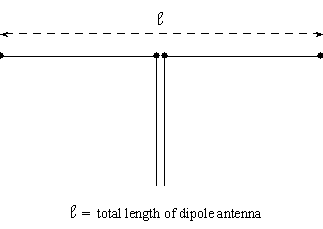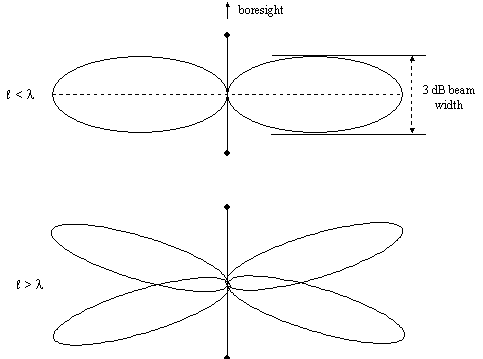# Dipole Antenna Pattern

Dipole antennas are modeled analytically, using modeling equations that can be found in standard antenna texts.

See, e.g., Balanis, Constantine, Antenna Theory: Analysis and Design, New York: Wiley (1982), chapter 4, and Johnson, Richard C, Antenna Engineering Handbook, 3rd ed., New York: McGraw-Hill (1993), chapter 4.

The basic attributes of a dipole antenna pattern are illustrated in the following figure, in which the dimension labeled with a script l represents the length of the antenna:Dipole antenna pattern

Dipole radiation is lateral to the axis of the antenna and is simulated for a wide range of antenna lengths, from values less than the wavelength of the signal to values several times the wavelength.

Since the radiation is lateral to the antenna axis, the gain null points are along the antenna boresight.

As illustrated in the following figure, if the antenna length is less than the wavelength of the signal, a single sidelobe is generated in each direction.Dipole radiation and antenna length/wavelength ratio

The antenna's 3 dB beam width is proportionate to its length in this case. Yet, if the antenna length exceeds the wavelength, the sidelobe splits one or more times and the 3 dB beamwidth is not predictable in the present model.

Only farfield radiation conditions are modeled here; nearfield radiation effects are not simulated. Also, small diameter (thin wire) antennas are assumed, and effects stemming from larger antenna diameters are not modeled.

This antenna uses a polar coordinate system.

When you select this antenna type, the following parameters need to be specified:

Field Description
Design Frequency

The frequency of the antenna. The antenna design frequency is independent of the operational frequency of a transmitter, receiver, or radar. Changing the frequency of a transmitter, receiver, or radar does not update an embedded antenna's design frequency, or vice versa. The design frequency is solely used at antenna configuration time to compute the antenna size from its max gain or beamwidth settings. A mismatch between signal frequency and antenna design frequency typically causes performance degradation.

Length The total length of the dipole antenna (that is, the dimension designated byin the illustration above).
Length/Wave Length Ratio The ratio of length () to wave length (lambda). Modifying this value changes the length of the Dipole antenna.
Efficiency The efficiency factor of the antenna, varying from 0 to 100 percent. Since no antenna is perfect, the efficiency factor allows you to specify the degradation in performance. Typical values fall in the range of 70 to 90 percent.

Make certain that the value you enter for Length represents the TOTAL length of the dipole antenna, NOT the length of one of its two segments. Otherwise, the results will not be what you expect. If you use the default frequency of 14.5 GHz for a communications receiver or transmitter (instead of a frequency in the usual range for dipole operation), make certain to adjust the Length accordingly. For example, you might wish to use a Length of 0.02 m for single sidelobes or 0.03 m for split sidelobes. Also, if you use the Az-El orientation method, the elevation should be set at 0 deg instead of the default value of 90 deg. The default antenna length of 1.00 m is good for frequencies ranging between 300 and 1000 MHz.